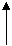MY FIRST C++ PROGRAM

Introduction to C++

C++ is an object oriented language, which combines the best of the structured programming techniques of C, thus making it a very powerful language. C++ is based on C and Simula 67 language. C was invented by Bjarne Stroustrup.

Since C++ is a superset of C, therefore most of C language constructs apply in C++ as well. A program in C++ can be written in both C style and Object Oriented style.

First Program

Let us start our tour of C++ with a simple program. Here is a simple program which outputs a line of text. It’s output is shown in the right column.

 // This is my first C++ program //It prints a line of text # include int main() { std:: cout << “My first C++ program ” << std::endl; return 0;   } My first C++ program

Structure of the C++ Program and C++ features

Above program demonstrates several important features of C++ language. The structure of the program above is as follows:-

// This is my first C++ program

//It prints a line of text

# include <iostream>

is a preprocessor directive. It tells the preprocessor to include the contents of iostream header file in the program before compilation. This file is required for input output statements.

main Function

int main()

is a function. C++ program is a collection of functions. Every program in C++ begins executing at the function main(). Every C++ program has exactly one main function and a collection of other functions.

A function is the main entity where all of the functionality of a program is defined. A function takes some input, processes it according to the some code written in the function body and produces an output which can be either sent to the user or can be used by other functions.

Keyword int in int main()

specifies the return type of a function. int specifies that the function main returns an integer value.

Brackets () after main signify that main is a function. Every function should be followed by a pair of brackets. The purpose of brackets is to pass the parameters list to the functions. Parameters are the number and type of arguments (inputs) which a function takes.

Opening brace ( { ) and closing brace ( } ) mark the beginning and end of a function. Anything which is inside the braces is the body of that function.

In our example, the function body consists of only two statements,

{

std::cout << “My first C++ program. \n”;

return 0;

}

Statement

std::cout << “My first C++ program ” << std::endl;

The above line is a statement in C++. A statement must always terminate with a semicolon (;) otherwise it causes a syntax error. This statement introduces two new features of C++ language, cout and << operator.

When this statement is executed, it sends the string between the quotation marks to the standard output stream objectcout. cout is a predefined object in C++ and the standard output stream is normally the screen.

The purpose of standard output stream object is to print the output on the device to which it is connected. Normally, the output device is screen but it can be programmed to output to any other device such as a printer. The following diagram illustrated the concept of cout.

User Screen

MonitorObject                              Insertion Operator                                 String variable

We used std:: before cout. This is required when we use # include <iostream> .

It specifies that we are using a name (cout) which belongs to namespace std. Namespace is a new concept introduced by ANSI C++ which defines the scope of identifiers which are used in the program. std is the namespace where C++ standard libraries are defined.

Operator << is the insertion stream operator. It sends contents of variable on its right to the object on its left. In our case, right operand is the string “My first C++ program” and left operand is cout object. So it sends the string to the cout object and cout object then displays it on the output screen.

endl is the line feed operator in C++. It acts as a stream manipulator whose purpose is to feed the whole line and then point the cursor to the beginning of the next line. We can use \n (\n is an escape sequence) instead of endl for the same purpose. Then the code would be

std::cout << “My first C++ program \n”;

Return Statement

Last line in the body of our first program is

return 0;

The keyword return is a special statement which is used to exit a function and return a value. This statement exits the function main indicating its completion and returns the value 0 to the operating system indicating that the program successfully ran to completion. return statement is included at the end of every main function.

OUTPUT

When this program is run, it prints the following output

My first C++ program

For programs to do exciting things, they should be written, compiled and then run on a computer using a compile or IDE. Next tutorial, How to compile and run programs shows how to run programs.

Congratulations!! You have just run your first C++ program and have understood many important features of C++.

Proceed to the next lesson